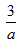小学教育 >> 五年级 >> 数学下 >> 内容
 五年级数学解方程专项练习题（附知识点）

 本信息由 系统管理员 于 05-01 10:13 发布 共211次访问
 五年级数学解方程（专项练习） 1、知识点：1、用字母表示数（1）用字母表示数量关系（2）用字母表示计算公式（3）用字母表示运算定律和计算法则（4）求代数式的值：把给定字母的数值代入式子，求出式子的值。 2、注意：（1）数字和字母、字母和字母相乘时，乘号可以记作“·”，或者省略不写，数字要写在字母的前面。 （2）当1与任何字母相乘时，1省略不写。（3）在一个问题中，不同的量用不同的字母来表示，而不能用同一个字母表示。（4）字母可以表示任意数，所以在一些式子中，对字母的表示要进行说明。如：（a≠0） 3、简易方程：（1）方程：含有未知数的等式叫作方程。方程都是等式，等式不一定是方程，只有当等式中含有未知数时，才是方程。（2）方程的解：使方程左右两边相等的未知数的值叫作方程的解。（3）解方程：求方程的解的过程叫作解方程。（4）方程的解是一个值，一般来说，没有解方程这个计算过程，方程的解是难以求出的，解方程是求方程的解的过程，是一个演算过程。一、基础类方程。x-7.7=2.85     5x-3x=68       4x+10=18  321=45+6x     x-0.6x=8       x+8.6=9.4    52－2x＝15    13÷x ＝1.3     x+8.3=19.7  15x ＝30        3x+9＝36       7(x-2)=7    3x+9=12       18(x-2)=27      12x=320+4x    5.37+x=7.47      15÷3x=5      30÷x=75    1.8+2x=6       420-3x=180      3(x+5)=18 0.5x+9=40      6x+3x=36        1.5x+6=3x  5×3-x=8         40-8x=5           x÷5=21 48-20+5x=31      x+2x+8=80    200-x÷5=30 70÷x=4     45.6- 3x =0.6       9.8-2x=3.8  5(x+5)=100      x+3x=70      2.5(x+3)=50  二、提高类方程。3（4x－1）=3（22－x）   7（2x-6）=84 5（x-8）=3x        7x-7=6x+4 （22-x）+2=87x      8x-6x+30=12x+15 7（x+2）=5x+60      240÷（x－7）=30   （31-8x）÷3=2x+1      （6x-28）÷8=5x-8 12÷8x=3         （21+4x）×2=10x+14 8x-15×6=3x-20      （2x+7）×2=3x+18

 【加入收藏】 【打印此页】 【关闭本页】数字校园门户网站  冀ICP备  号 旗舰版 V3.0     技术支持：邢台力合文化传播有限公司 Copyright © 数字校园管理平台